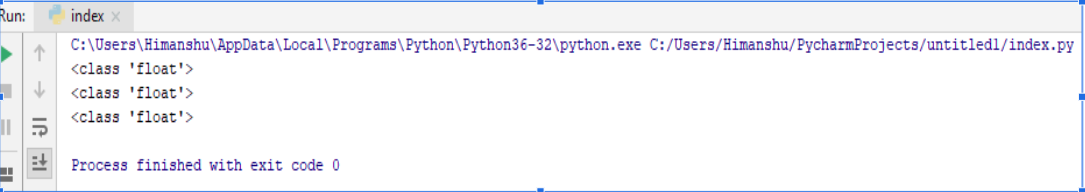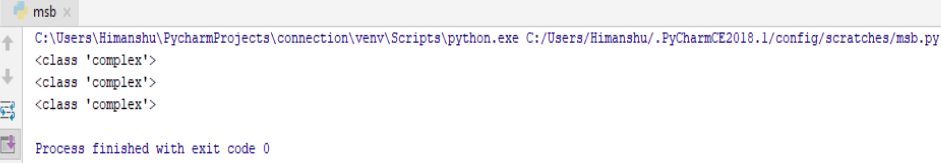×

Html & Html5

Introduction to Html

Component of Html

Html Form

Html5 basics

Html5 Grid

Html5 Graphics

Html5 Media

Html5 APIs

Python and Pandas

Introduction to Python

Component of Python

Python Numbers

In Python there are three type of numerics . These are as follows :-

• int
• float
• complex

Numeric int

Integer or int includes infinite - natural numbers , whole numbers , positive or negative numbers without decimal .
So let’s see...

a=50

b=46464646746413164613215

c=-6346432886585858585

print(type(a))

print(type(b))

print(type(c))

Output of this Program

Here in the above program, first we have assigned the different values to different variables , than through Python command we ask to Python interpreter to just tell us the type of the variable .
So here comes the output :-And it just tells us that , it is ‘int’ type variable.

Numeric float

Float basically contains positive or negative numbers including decimals.
For example:-

a = 45.1

b=65.87

c=35.21443131464313130

print(type(a))

print(type(b))

print(type(c))

Output of this Program

Here in the above program, first we have assigned the different decimal values to different variables , than through Python command we ask to Python interpreter to just tell us the type of the variable .
So here comes the output :-And it just tell us that it is a ‘float’ type variable.

Numeric complex

Complex basically contains ‘j’ as the imaginary part.
For example :-

a=3+9j

b=16j

c=-24j

print(type(a))

print(type(b))

print(type(c))

Output of this Program

Here in the above program, first we have assigned the different values to different variables including ‘j’ also which is an imaginary part , than through Python command we ask to Python interpreter to just tell us the type of the variable .
So here comes the output :-And it just tell us that it is a ‘complex’ type variable.

python training insitute| Best IT Training classes in Gurgaon | python training